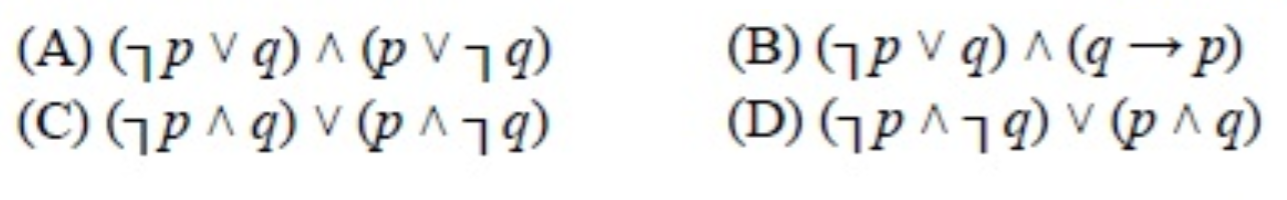Related Articles

# GATE | GATE-CS-2015 (Set 1) | Question 65

• Difficulty Level : Easy
• Last Updated : 07 Sep, 2018

Which one of the following is not equivalent to p←→q(A) A
(B) B
(C) C
(D) D

Explanation: This question is the revision of basics of propositional logic.
Conjunction of p and q, denoted by p∧q, is the proposition ‘p and q’. The conjunction p ∧ q is True, when both p and q is True.

Disjunction of p and q, denoted by p∨q, is the proposition ‘p or q’. The disjunction p∨q is False when both p and q is False.

Logical Implication –
It is a type of relationship between two statements or sentence. Denoted by ‘p → q’. The
conditional statement p → q is false when p is true and q is false, and true otherwise.
i.e. p → q = ¬p ∨ q

Bi-Condition
A bi-conditional statement is a compound statement formed by combining two conditionals under
“and.” Bi-conditionals are true when both statements have the exact same truth value.

Solution :
p↔q means both p→q and q→p
p→q is equivalent to ⌉p ∨ q and q is equivalent to ⌉q ∨ p
So A and B are fine.
D is a different way of writing A
p ↔ q = (p→ q) ∧ (q→p)
= (⌉p ∨ q) ∧ (q → p) [ Since p→ q = ⌉p ∨ q ]
= (⌉p ∨ q) ∧ (⌉q ∨ p)
= (¬p ∧ p )∨ (¬p ∧¬q )∨ (q ∧p ) ∨(q ∧¬q ) (Distributive law)
[As ((¬p∧ p )=0,(q ∧¬q )=0) (Complementation) ]
(⌉p ∧ ⌉q) ∨ (p ∧ q) which is Option (D)
Only option which is not equivalent to p↔q is option (C).

So, option (C) is correct.

Quiz of this Question

Attention reader! Don’t stop learning now.  Practice GATE exam well before the actual exam with the subject-wise and overall quizzes available in GATE Test Series Course.

Learn all GATE CS concepts with Free Live Classes on our youtube channel.

My Personal Notes arrow_drop_up